0
213

# CAT Linear and Circular Arrangement Questions [PDF]

Arragements is a very important topic in the CAT LRDI Section. Linear & Circular Arrangement questions are not very difficult if you represent the data properly. Sets on Arrangement questions are asked frequently in the CAT. You can expect a set from Arrangements in the latest format of the CAT LRDI section. This article will look into some interesting Arrangement Questions for the CAT LRDI section. If you want to practice these important Linear & Circular Arrangement questions, you can download the PDF, which is completely Free.

Instructions

DIRECTIONS for the following three questions: Answer the questions on the basis of the information given below.

Five friends meet every morning at Sree Sagar restaurant for an idli-vada breakfast. Each consumes a different number of idlis and vadas. The number of idlis consumed are 1, 4, 5, 6, and 8, while the number of vadas consumed are 0, 1, 2, 4, and 6. Below are some more facts about who eats what and how much.

i. The number of vadas eaten by Ignesh is three times the number of vadas consumed by the person who eats four idlis.

ii. Three persons, including the one who eats four vadas eat without chutney.

iii. Sandeep does not take any chutney.

iv. The one who eats one idli a day does not eat any vadas or chutney. Further, he is not Mukesh.

v. Daljit eats idli with chutney and also eats vada.

vi. Mukesh, who does not take chutney, eats half as many vadas as the person who eats twice as many idlis as he does.

vii. Bimal eats two more idlis than Ignesh, but Ignesh eats two more vadas than Bimal.

Question 1: Which one of the following statements is true?

a) Daljit eats 5 idlis

b) Ignesh eats 8 idlis

c) Bimal eats 1 idli.

d) Bimal eats 6 idlis.

Solution:

Considering (i), Ignesh has to eat 6 vadas, since 6 is the only multiple of 3.

Also, using the same information, we can say that a person consumes 2 vadas and 4 idlis.

Using (vii), Bimal eats 2 more idlis than Ignesh.

Possibilities:

Bimal – 8, Ignesh – 6

Bimal – 6, Ignesh – 4

But Ignesh cannot have 4 idlis because the person who eats 4 idlis eats 2 vadas.

Hence we take Bimal – 8 and Ignesh – 6.

Also, we get that Bimal eats 6 – 2 = 4 vadas.

So far, we get the following information.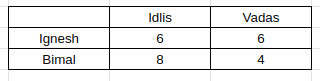Using (vi), there is a person who eats twice as many idlis as Mukesh. The only pair satisfying is 8, 4.

So, Mukesh eats 4 idlis. Plus the person who eats 4 idlis eats 2 vadas. Hence, we get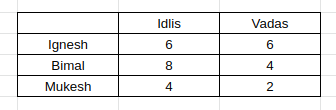Daljit also eats Vada as per info (v), so we get the following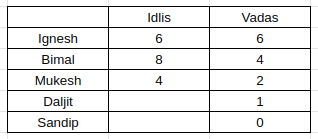(iv) gives us the information that the one who eats 1idli does not have vada.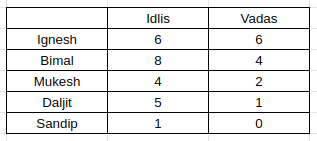Considering the persons who had chutney and those who didn’t, 3 persons do not have chutney. Bimal is one of them(the one eating 4 vadas).

Mukesh is the second one to not take chutney(last hint). Also, Sandip does not take chutney. Hence, we get this information as well.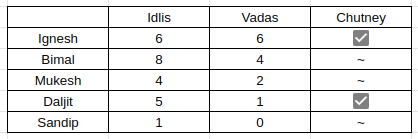Option A, stating that Daljit eats 5 idlis, is right.

Question 2: Which of the following statements is true?

Solution:

Considering (i), Ignesh has to eat 6 vadas, since 6 is the only multiple of 3.

Also, using the same information, we can say that a person consumes 2 vadas and 4 idlis.

Using (vii), Bimal eats 2 more idlis than Ignesh.

Possibilities:

Bimal – 8, Ignesh – 6

Bimal – 6, Ignesh – 4

But Ignesh cannot have 4 idlis because the person who eats 4 idlis eats 2 vadas.

Hence we take Bimal – 8 and Ignesh – 6.

Also, we get that Bimal eats 6 – 2 = 4 vadas.

So far, we get the following information.Using (vi), there is a person who eats twice as many idlis as Mukesh. The only pair satisfying is 8, 4.

So, Mukesh eats 4 idlis. Plus the person who eats 4 idlis eats 2 vadas. Hence, we getDaljit also eats Vada as per info (v), so we get the following(iv) gives us the information that the one who eats 1idli does not have vada.Considering the persons who had chutney and those who didn’t, 3 persons do not have chutney. Bimal is one of them(the one eating 4 vadas).

Mukesh is the second one to not take chutney(last hint). Also, Sandip does not take chutney. Hence, we get this information as well.Question 3: Which of the following statements is true?

a) Mukesh eats 8 idlis and 4 vadas but no chutney.

b) The person who eats 5 idlis and 1 vada does not take chutney.

c) The person who eats equal number of vadas and idlis also takes chutney.

d) The person who eats 4 idlis and 2 vadas also takes chutney.

Solution:

Considering (i), Ignesh has to eat 6 vadas, since 6 is the only multiple of 3.

Also, using the same information, we can say that a person consumes 2 vadas and 4 idlis.

Using (vii), Bimal eats 2 more idlis than Ignesh.

Possibilities:

Bimal – 8, Ignesh – 6

Bimal – 6, Ignesh – 4

But Ignesh cannot have 4 idlis because the person who eats 4 idlis eats 2 vadas.

Hence we take Bimal – 8 and Ignesh – 6.

Also, we get that Bimal eats 6 – 2 = 4 vadas.

So far, we get the following information.Using (vi), there is a person who eats twice as many idlis as Mukesh. The only pair satisfying is 8, 4.

So, Mukesh eats 4 idlis. Plus the person who eats 4 idlis eats 2 vadas. Hence, we getDaljit also eats Vada as per info (v), so we get the following(iv) gives us the information that the one who eats 1idli does not have vada.Considering the persons who had chutney and those who didn’t, 3 persons do not have chutney. Bimal is one of them(the one eating 4 vadas).

Mukesh is the second one to not take chutney(last hint). Also, Sandip does not take chutney. Hence, we get this information as well.Hence, Ignesh eating 6 vadas and 6 idlis eat Chutney.

Question 4: Eight people carrying food baskets are going for a picnic on motorcycles.
Their names are A, B, C, D, E, F, G, and H. They have 4 motorcycles M1, M2, M3 and M4 among them. They also have 4 food baskets O, P, Q and R of different sizes and shapes and each can be carried only on motorcycles M1, M2, M3 and M4 respectively.
No more than 2 persons can travel on a motorcycle and no more than one basket can be carried on a motorcycle. There are 2 husband-wife pairs in this group of 8 people and each pair will ride on a motorcycle together.
C cannot travel with A or B. E cannot travel with B or F. G cannot travel with F, or H, or D.
The husband-wife pairs must carry baskets O and P. Q is with A and P is with D.
F travels on M1 and E travels on M2 motorcycles.
G is with Q, and B cannot go with R.
Who is travelling with H?

a) A

b) B

c) C

d) D

Solution:

It is given in the statements, that C cannot travel with A or B. E cannot travel with B or F. G cannot travel with F, or H, or D. By formulating the table we get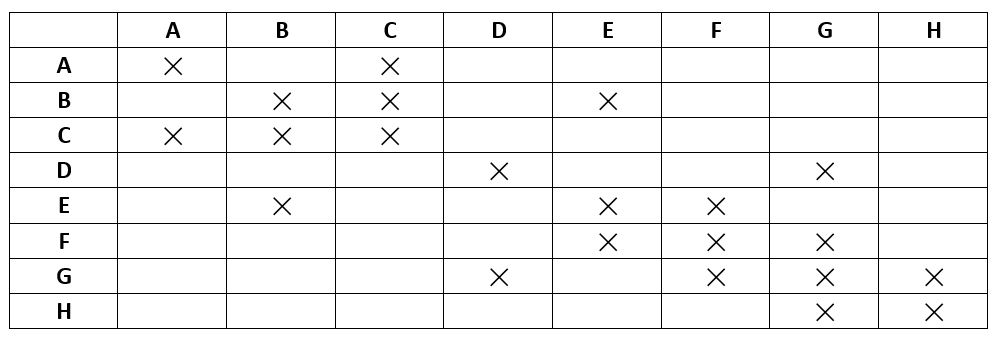Q is with A and G is with Q => G and Q are travelling together on motorcycle M3
F travels on M1 and E travels on M2 motorcycles.
D is travelling with P on M2 => D and E are traveling together on M2
B cannot go with R => F and B go together on M1
Therefore, C and H go together on M4

So, the table can formed as below :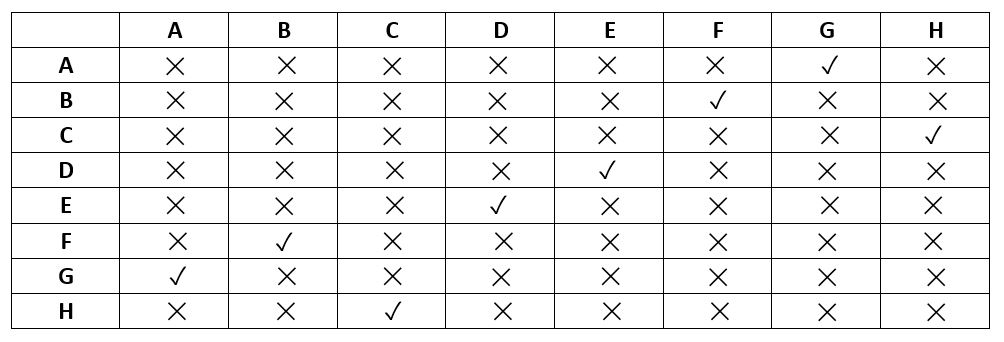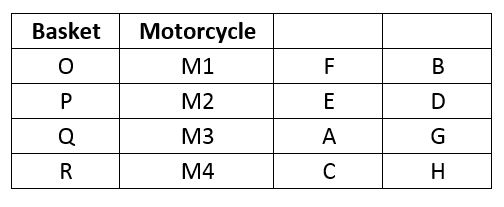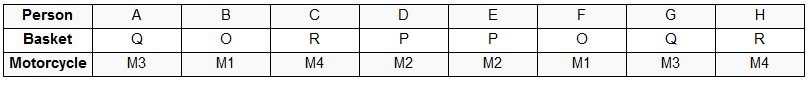Hence, C would be travelling with H.

Question 5: Abraham, Border, Charlie, Dennis and Elmer and their respective wives recently dined together and were seated at a circular table. The seats were so arranged that men and women alternated and each woman was three places distant from her husband. Mrs. Charlie sat to the immediate left of Mr. Abraham. Mrs. Elmer sat two places to the right of Mrs. Border. Who sat to the right of Mr. Abraham?

a) Mrs. Dennis

b) Mrs. Elmer

c) Mrs. Border

d) Mrs. Border or Mrs. Dennis

Solution:

Mrs. Abraham can’t be sitting next to him as per the seating arrangement. Wives sit three places away from their husbands.

Mrs. Charlie is sitting to the left of Mr. Abraham. So, she can’t be sitting to his right.

Mrs. Elenor is sitting two places to the right of Mrs. Border (and not Mrs. Charlie). So, she can’t be sitting right next to Mr. Abraham.

Mrs. Border and Mrs. Dennis are the remaining two wives and each is equally likely to to be sitting to the right of Mr. Abraham.

Instructions

There were seven elective courses – E1 to E7 – running in a specific term in a college. Each of the 300 students enrolled had chosen just one elective from among these seven. However, before the start of the term, E7 was withdrawn as the instructor concerned had left the college. The students who had opted for E7 were allowed to join any of the remaining electives. Also, the students who had chosen other electives were given one chance to change their choice. The table below captures the movement of the students from one elective to another during this process. Movement from one elective to the same elective simply means no movement. Some numbers in the table got accidentally erased; however, it is known that these were either 0 or 1.

Further, the following are known:
1. Before the change process there were 6 more students in E1 than in E4, but after the reshuffle, the number of students in E4 was 3 more than that in E1.
2. The number of students in E2 increased by 30 after the change process.
3. Before the change process, E4 had 2 more students than E6, while E2 had 10 more students than E3.

Question 6: How many elective courses among E1 to E6 had a decrease in their enrollments after the change process?

a) 4

b) 1

c) 2

d) 3

Solution:

From the table we can say that number of students who opted for E2 after reshuffle = 5 + 34 + 6 + 3 + 5 + 7 + 16 = 76.

It is given us that the number of students in E2 increased by 30 after the change process. Hence, we can say that the number of students who were enrolled in E2 before reshuffle = 76 – 30 = 46.

It is given that before the change process there were 10 more students in E2 than in E3. Therefore, the number of students who were enrolled in E3 before reshuffle = 46 – 10 = 36.

Number of students who moved from E1 to all other electives are known. Therefore, the number of students who were enrolled in E1 before reshuffle =  9 + 5 + 10 + 1 + 4 + 2 = 31.

It is given that before the change process there were 6 more students in E1 than in E4. Therefore, the number of students who were enrolled in E4 before reshuffle = 31 – 6 = 25.

Also, it is given that E4 had 2 more students than E6 before reshuffle. Therefore, the number of students who were enrolled in E6 before reshuffle =  25 – 2 = 23.

All the students from E7 moved to one of electives among E1 to E6.
Therefore, the number of students who were enrolled in E7 before reshuffle = 4 + 16 + 30 + 5 + 5 + 41 = 101.

Except E5 we know the number of students who were enrolled in all electives. We also know that there were total 300 students who opted for exactly 1 elective.

Hence, the the number of students who were enrolled in E7 before reshuffle = 300 – (46+36+31+25+23+101) = 38.

For each elective, the number of students who were enrolled before reshuffle will be same as sum of the number of students who moved from that elective to another elective including no movement cases.

For elective E2,

Number of students who moved to E1 + 34 + 8 + Number of students who moved to E4 + 2 + 2 = 46

i.e. Number of students who moved from to E1 =  Number of students who moved from to E4 = 0

For elective E4,

Number of students who moved to E1 + 3 + 2 + 14 + Number of students who moved to E5 + 4 = 25

i.e. Number of students who moved from to E1 =  Number of students who moved from to E5 = 1     {As the remaining blanks can be filled by either 0 or 1}

For elective E6,

Number of students who moved to E1 + 7 + 3 + Number of students who moved to E4 + 2 + 9 = 23

i.e. Number of students who moved from to E1 =  Number of students who moved from to E4 = 1     {As the remaining blanks can be filled by either 0 or 1}

It is given that after the reshuffle, the number of students in E4 was 3 more than that in E1.
As of now the number of students enrolled in E4 after reshuffle = 1 + 0 + E3 to E4 + 14 +  E5 to E4 + 1 + 5 = 21 + {E3 to E4} + {E5 to E4}

Also, the number of students enrolled in E1 after reshuffle = 9 + 0 + 2 + 1 + E5 to E1 + 1 + 4  = 17 + E5 to E1.

Hence, it is possible only when  E5 to E1 = 1 and E3 to E4 = E5 to E4 = 0.

Remaining blank places can be filled easily as we know the total sum of each row.

Therefore, the number of students who moved from E3 to E5 = the number of students who moved from E5 to E3 = the number of students who moved from E5 to E6 = 1.

Form the table we can see that the number of students who enrolled for E1 and E4 decreased from 31 and 25 to 18 and 21 respectively.

Therefore, option C is the correct answer.

Question 7: After the change process, which of the following is the correct sequence of number of students in the six electives E 1 to E6?

a) 19, 76, 79, 21, 45, 60

b) 19, 76, 78, 22, 45, 60

c) 18, 76, 79, 23, 43, 61

d) 18, 76, 79, 21, 45, 61

Solution:

From the table we can say that number of students who opted for E2 after reshuffle = 5 + 34 + 6 + 3 + 5 + 7 + 16 = 76.

It is given us that the number of students in E2 increased by 30 after the change process. Hence, we can say that the number of students who were enrolled in E2 before reshuffle = 76 – 30 = 46.

It is given that before the change process there were 10 more students in E2 than in E3. Therefore, the number of students who were enrolled in E3 before reshuffle = 46 – 10 = 36.

Number of students who moved from E1 to all other electives are known. Therefore, the number of students who were enrolled in E1 before reshuffle =  9 + 5 + 10 + 1 + 4 + 2 = 31.

It is given that before the change process there were 6 more students in E1 than in E4. Therefore, the number of students who were enrolled in E4 before reshuffle = 31 – 6 = 25.

Also, it is given that E4 had 2 more students than E6 before reshuffle. Therefore, the number of students who were enrolled in E6 before reshuffle =  25 – 2 = 23.

All the students from E7 moved to one of electives among E1 to E6.
Therefore, the number of students who were enrolled in E7 before reshuffle = 4 + 16 + 30 + 5 + 5 + 41 = 101.

Except E5 we know the number of students who were enrolled in all electives. We also know that there were total 300 students who opted for exactly 1 elective.

Hence, the the number of students who were enrolled in E7 before reshuffle = 300 – (46+36+31+25+23+101) = 38.

For each elective, the number of students who were enrolled before reshuffle will be same as sum of the number of students who moved from that elective to another elective including no movement cases.

For elective E2,

Number of students who moved to E1 + 34 + 8 + Number of students who moved to E4 + 2 + 2 = 46

i.e. Number of students who moved from to E1 =  Number of students who moved from to E4 = 0

For elective E4,

Number of students who moved to E1 + 3 + 2 + 14 + Number of students who moved to E5 + 4 = 25

i.e. Number of students who moved from to E1 =  Number of students who moved from to E5 = 1     {As the remaining blanks can be filled by either 0 or 1}

For elective E6,

Number of students who moved to E1 + 7 + 3 + Number of students who moved to E4 + 2 + 9 = 23

i.e. Number of students who moved from to E1 =  Number of students who moved from to E4 = 1     {As the remaining blanks can be filled by either 0 or 1}

It is given that after the reshuffle, the number of students in E4 was 3 more than that in E1.
As of now the number of students enrolled in E4 after reshuffle = 1 + 0 + E3 to E4 + 14 +  E5 to E4 + 1 + 5 = 21 + {E3 to E4} + {E5 to E4}

Also, the number of students enrolled in E1 after reshuffle = 9 + 0 + 2 + 1 + E5 to E1 + 1 + 4  = 17 + E5 to E1.

Hence, it is possible only when  E5 to E1 = 1 and E3 to E4 = E5 to E4 = 0.

Remaining blank places can be filled easily as we know the total sum of each row.

Therefore, the number of students who moved from E3 to E5 = the number of students who moved from E5 to E3 = the number of students who moved from E5 to E6 = 1.

Form the table, we can see that after the reshuffle the number of students in electives E1 to E6 are 18, 76, 79, 21, 45 and 61 in that order.

Therefore, option D is the correct answer.

Question 8: After the change process, which course among E1 to E6 had the largest change in its enrollment as a percentage of its original enrollment?

a) E1

b) E2

c) E3

d) E6

Solution:

From the table we can say that number of students who opted for E2 after reshuffle = 5 + 34 + 6 + 3 + 5 + 7 + 16 = 76.

It is given us that the number of students in E2 increased by 30 after the change process. Hence, we can say that the number of students who were enrolled in E2 before reshuffle = 76 – 30 = 46.

It is given that before the change process there were 10 more students in E2 than in E3. Therefore, the number of students who were enrolled in E3 before reshuffle = 46 – 10 = 36.

Number of students who moved from E1 to all other electives are known. Therefore, the number of students who were enrolled in E1 before reshuffle =  9 + 5 + 10 + 1 + 4 + 2 = 31.

It is given that before the change process there were 6 more students in E1 than in E4. Therefore, the number of students who were enrolled in E4 before reshuffle = 31 – 6 = 25.

Also, it is given that E4 had 2 more students than E6 before reshuffle. Therefore, the number of students who were enrolled in E6 before reshuffle =  25 – 2 = 23.

All the students from E7 moved to one of electives among E1 to E6.
Therefore, the number of students who were enrolled in E7 before reshuffle = 4 + 16 + 30 + 5 + 5 + 41 = 101.

Except E5 we know the number of students who were enrolled in all electives. We also know that there were total 300 students who opted for exactly 1 elective.

Hence, the the number of students who were enrolled in E7 before reshuffle = 300 – (46+36+31+25+23+101) = 38.

For each elective, the number of students who were enrolled before reshuffle will be same as sum of the number of students who moved from that elective to another elective including no movement cases.

For elective E2,

Number of students who moved to E1 + 34 + 8 + Number of students who moved to E4 + 2 + 2 = 46

i.e. Number of students who moved from to E1 =  Number of students who moved from to E4 = 0

For elective E4,

Number of students who moved to E1 + 3 + 2 + 14 + Number of students who moved to E5 + 4 = 25

i.e. Number of students who moved from to E1 =  Number of students who moved from to E5 = 1     {As the remaining blanks can be filled by either 0 or 1}

For elective E6,

Number of students who moved to E1 + 7 + 3 + Number of students who moved to E4 + 2 + 9 = 23

i.e. Number of students who moved from to E1 =  Number of students who moved from to E4 = 1     {As the remaining blanks can be filled by either 0 or 1}

It is given that after the reshuffle, the number of students in E4 was 3 more than that in E1.
As of now the number of students enrolled in E4 after reshuffle = 1 + 0 + E3 to E4 + 14 +  E5 to E4 + 1 + 5 = 21 + {E3 to E4} + {E5 to E4}

Also, the number of students enrolled in E1 after reshuffle = 9 + 0 + 2 + 1 + E5 to E1 + 1 + 4  = 17 + E5 to E1.

Hence, it is possible only when  E5 to E1 = 1 and E3 to E4 = E5 to E4 = 0.

Remaining blank places can be filled easily as we know the total sum of each row.

Therefore, the number of students who moved from E3 to E5 = the number of students who moved from E5 to E3 = the number of students who moved from E5 to E6 = 1.

We are asked the largest change in its enrollment as a percentage of its original enrollment for all 6 electives but as we can see there are only 4 electives. Hence, we will check only for E1, E2, E3 and E6.

The percentage change in the number of students for E1 = $\dfrac{18-31}{31}\times 100$ $\approx$ 42 %

The percentage change in the number of students for E2 = $\dfrac{76-46}{46}\times 100$ $\approx$ 65 %

The percentage change in the number of students for E3 = $\dfrac{79-36}{36}\times 100$ $\approx$ 119 %

The percentage change in the number of students for E6 = $\dfrac{61-23}{23}\times 100$ $\approx$ 165 %

We can see that the percent change in the number of student for E6 is the largest. Therefore, option D is the correct answer.

Question 9: Later, the college imposed a condition that if after the change of electives, the enrollment in any elective (other than E7) dropped to less than 20 students, all the students who had left that course will be required to re-enroll for that elective.
Which of the following is a correct sequence of electives in decreasing order of their final enrollments?

a) E2, E3, E6, E5, E1, E4

b) E3, E2, E6, E5, E4, E1

c) E2, E5, E3, E1, E4, E6

d) E2, E3, E5, E6, E1, E3

Solution:

From the table we can say that number of students who opted for E2 after reshuffle = 5 + 34 + 6 + 3 + 5 + 7 + 16 = 76.

It is given us that the number of students in E2 increased by 30 after the change process. Hence, we can say that the number of students who were enrolled in E2 before reshuffle = 76 – 30 = 46.

It is given that before the change process there were 10 more students in E2 than in E3. Therefore, the number of students who were enrolled in E3 before reshuffle = 46 – 10 = 36.

Number of students who moved from E1 to all other electives are known. Therefore, the number of students who were enrolled in E1 before reshuffle =  9 + 5 + 10 + 1 + 4 + 2 = 31.

It is given that before the change process there were 6 more students in E1 than in E4. Therefore, the number of students who were enrolled in E4 before reshuffle = 31 – 6 = 25.

Also, it is given that E4 had 2 more students than E6 before reshuffle. Therefore, the number of students who were enrolled in E6 before reshuffle =  25 – 2 = 23.

All the students from E7 moved to one of electives among E1 to E6.  Therefore, the number of students who were enrolled in E7 before reshuffle = 4 + 16 + 30 + 5 + 5 + 41 = 101.

Except E5 we know the number of students who were enrolled in all electives. We also know that there were total 300 students who opted for exactly 1 elective.

Hence, the the number of students who were enrolled in E7 before reshuffle = 300 – (46+36+31+25+23+101) = 38.

For each elective, the number of students who were enrolled before reshuffle will be same as sum of the number of students who moved from that elective to another elective including no movement cases.

For elective E2,

Number of students who moved to E1 + 34 + 8 + Number of students who moved to E4 + 2 + 2 = 46

i.e. Number of students who moved from to E1 =  Number of students who moved from to E4 = 0

For elective E4,

Number of students who moved to E1 + 3 + 2 + 14 + Number of students who moved to E5 + 4 = 25

i.e. Number of students who moved from to E1 =  Number of students who moved from to E5 = 1     {As the remaining blanks can be filled by either 0 or 1}

For elective E6,

Number of students who moved to E1 + 7 + 3 + Number of students who moved to E4 + 2 + 9 = 23

i.e. Number of students who moved from to E1 =  Number of students who moved from to E4 = 1     {As the remaining blanks can be filled by either 0 or 1}

It is given that after the reshuffle, the number of students in E4 was 3 more than that in E1. As of now the number of students enrolled in E4 after reshuffle = 1 + 0 + E3 to E4 + 14 +  E5 to E4 + 1 + 5 = 21 + {E3 to E4} + {E5 to E4}

Also, the number of students enrolled in E1 after reshuffle = 9 + 0 + 2 + 1 + E5 to E1 + 1 + 4  = 17 + E5 to E1.

Hence, it is possible only when  E5 to E1 = 1 and E3 to E4 = E5 to E4 = 0.

Remaining blank places can be filled easily as we know the total sum of each row.

Therefore, the number of students who moved from E3 to E5 = the number of students who moved from E5 to E3 = the number of students who moved from E5 to E6 = 1.

We can see from the table that number of students enrolled in E1 dropped to 18. Hence, all the students who moved from E1 to any other elective will have to re-enroll in E1.

We can see that the number of students who enrolled for E1 prior to reshuffle = 31. Out of these 31 students, 9 students didn’t move to any other elective whereas remaining 22 students moved to other electives. Hence, all these 22 students have to re-enroll in E1.

Therefore, the total number of students in E1 post re-enrollment = 18 + 22 = 40 which is shown in the table.

Therefore, the sequence of electives in decreasing order of their final enrollments = E2, E3, E6, E5, E1, E4.

Hence, option A is the correct answer.

Instructions

At a management school, the oldest M dorms, numbered 1 to 10, need to be repaired urgently. This following diagram represents the estimated repair costs (in Rs. Crores for, the 10 dorms. For any dorm, the estimated repair cost (in Rs. Crores ) is an integer. Repairs with estimated cost Rs. 1 or 2 Crores are considered light repairs, repairs with estimated cost Rs. 3 or 4 are considered moderate repairs and repairs with estimated cost Rs. 5 or 6 Crores are considered extensive repairs.

Further, the following information is known.
1. Odd-numbered dorms do not need light repair; even-numbered dorms do not need moderate repair and dorms, whose numbers are divisible by 3, do not need extensive repair.
2. Dorms 4 to 9 all need different repair costs, with Dorm 7 needing the maximum and Dorm 8 needing the minimum.

Question 10: Which of the following is NOT necessarily true?

a) Dorm 1 needs a moderate repair

b) Dorm 5 repair will cost no more than Rs. 4 Crores

c) Dorm 7 needs an extensive repair

d) Dorm 10 repair will cost no more than Rs. 4 Crores

Solution:

Odd numbered dorms need either moderate or extensive repair.

Even numbered dorms need either light or extensive repair.

It has been given that dorms 4 to 9 all require different repairing costs. The dorms 3 and 9 should require moderate repair (going by the table). Dorm 7 costs the highest. Therefore, dorm 7 should require 6 crores to repair. Dorm 8 requires the least cost to repair. Therefore, dorm 8 should cost 1 crore to repair. We can eliminate these dorm numbers from other 2 lists.

Dorms 4 to 9 cost different costs to repair. => Both dorms 5 and 9 cannot require the same cost of repair. Dorms 1 and 3 should require 3 crores to repair.

Dorm 6 should require light repair (2 crores) since dorm 8 requires 1 crore to repair.
=> Dorm 4 requires 5 crore to repair.

We can see that all options except option D are definitely true. Option D cannot be ascertained to be true. Dorm 10 can cost Rs. 1 crore or Rs. 6 crores to repair. Therefore, option D is the right answer.

Question 11: What is the total cost of repairing the odd-numbered dorms (in Rs. Crores)?

Solution:

Odd numbered dorms need either moderate or extensive repair.

Even numbered dorms need either light or extensive repair.

It has been given that dorms 4 to 9 all require different repairing costs. The dorms 3 and 9 should require moderate repair (going by the table). Dorm 7 costs the highest. Therefore, dorm 7 should require 6 crores to repair. Dorm 8 requires the least cost to repair. Therefore, dorm 8 should cost 1 crore to repair. We can eliminate these dorm numbers from other 2 lists.

Dorms 4 to 9 cost different costs to repair. => Both dorms 5 and 9 cannot require the same cost of repair. Dorms 1 and 3 should require 3 crores to repair.

Dorm 6 should require light repair (2 crores) since dorm 8 requires 1 crore to repair.
=> Dorm 4 requires 5 crore to repair.

Cost = 3 + 3 + 6 + 3 + 4 = Rs.19 crores. Therefore, 19 is the correct answer.

Question 12: Suppose further that:
1. 4 of the 10 dorms needing repair are women’s dorms and need a total of Rs. 20 Crores for repair.
2. Only one of Dorms 1 to 5 is a women’s dorm.
What is the cost for repairing Dorm 9 (in Rs. Crores)?

Solution:

Odd numbered dorms need either moderate or extensive repair.

Even numbered dorms need either light or extensive repair.

It has been given that dorms 4 to 9 all require different repairing costs. The dorms 3 and 9 should require moderate repair (going by the table). Dorm 7 costs the highest. Therefore, dorm 7 should require 6 crores to repair. Dorm 8 requires the least cost to repair. Therefore, dorm 8 should cost 1 crore to repair. We can eliminate these dorm numbers from other 2 lists.

Dorms 4 to 9 cost different costs to repair. => Both dorms 5 and 9 cannot require the same cost of repair. Dorms 1 and 3 should require 3 crores to repair.

Dorm 6 should require light repair (2 crores) since dorm 8 requires 1 crore to repair.
=> Dorm 4 requires 5 crore to repair.

There are 3 dorms from 6 to 10 which are women’s dorms.

It has been given that the cost of repairing the woman dorms add up to 20. Therefore, the distribution of the costs should be 6+6+5+3.
Dorm 4 is the dorm whose number is below 5 but is a woman’s dorm. Therefore, dorm 9 should cost Rs.3 crores to repair. Dorm 8 cannot be a woman’s dorm. Therefore, dorm 10 should be a woman’s dorm and should cost Rs. 6 crore to repair.

Dorm 9 will cost Rs.9 crore to repair and hence, 9 is the correct answer.

Question 13: Suppose further that:
1. 4 of the 10 dorms needing repair are women’s dorms and need a total of Rs. 20 Crores for repair.
2. Only one of Dorms 1 to 5 is a women’s dorm.
Which of the following is a women’s dorm?

a) Dorm 2

b) Dorm 5

c) Dorm 8

d) Dorm 10

Solution:

Odd numbered dorms need either moderate or extensive repair.

Even numbered dorms need either light or extensive repair.

It has been given that dorms 4 to 9 all require different repairing costs. The dorms 3 and 9 should require moderate repair (going by the table). Dorm 7 costs the highest. Therefore, dorm 7 should require 6 crores to repair. Dorm 8 requires the least cost to repair. Therefore, dorm 8 should cost 1 crore to repair. We can eliminate these dorm numbers from other 2 lists.

Dorms 4 to 9 cost different costs to repair. => Both dorms 5 and 9 cannot require the same cost of repair. Dorms 1 and 3 should require 3 crores to repair.

Dorm 6 should require light repair (2 crores) since dorm 8 requires 1 crore to repair.
=> Dorm 4 requires 5 crore to repair.

It has been given that the cost of repairing the woman dorms add up to 20. Therefore, the distribution of the costs should be 6+6+5+3.
Dorm 4 is the dorm whose number is below 5 but is a woman’s dorm. Therefore, dorm 9 should cost Rs.3 crores to repair. Dorm 8 cannot be a woman’s dorm. Therefore, dorm 10 should be a woman’s dorm and should cost Rs. 6 crore to repair.

Hence, Option D is the right answer.

Instructions

A tea taster was assigned to rate teas from six different locations — Munnar, Wayanad, Ooty, Darjeeling, Assam and Himachal: These teas were placed in six cups, numbered 1 to 6, not necessarily in the same order. The tea taster was asked to rate these teas on the strength of their flavour on a scale of 1 to 10. He gave a unique integer rating to each tea. Some other information is given below:
a: Cup 6 contained tea from Himachal.
2. Tea from Ooty got the highest rating, but it was not in Cup 3.
3. The rating of tea in Cup 3 was double the rating of the tea in Cup 5.
4. Only two cups got ratings in even numbers.
5. Cup 2 got the minimum rating and this rating was an even number.
6. Tea in Cup 3 got a higher rating than that in Cup 1.
7. The rating of tea from Wayanad was more than the rating of tea from Munnar, but less than that from Assam.

Question 14: What was the second highest rating given?

Solution:

Now we are given that the lowest rating is an even number and only 2 cups got an even number rating.
Let’s take cases:-
1. The lowest rating is 4
If the lowest rating in 4 then the other ratings will be in the range 5-10.
From this we need 4 odd and 1 even numbers.
This is not possible as there are only 3 odd numbers from 5-10.
Thus, the lowest rating is not 4.
2. The lowest rating is 2.
If the lowest rating in 4 then the other ratings will be in the range 5-10.
From this we need 4 odd and 1 even numbers.
This is possible when the odd ratings are 3,5,7 and 9.
We are given that the highest rating is not even. Thus, 10 rating is not possible.
We are also given that the rating of tea in Cup 3 was double the rating of the tea in Cup 5.
Thus, the rating of the tea in cup 3 is an even number.
Thus, the rating of the tea in cup 5 must be an odd number.
Only 1 such pair is possible of 3 and 6.
Thus, the tea in cup 2 got the rating of 2.
The tea in cup 3 got a rating of 6 and the tea in cup 5 got a rating of 3.
We are given that:-
Tea in Cup 3 got a higher rating than that in Cup 1.
Thus, the tea in cup 1 got a rating of 5.
Cup 6 contained tea from Himachal and the Tea from Ooty got the highest rating.
Thus, cup 6 got a rating of 7 and cup 4 got a rating of 9.
The table is as shown below:-

Hence, 7 is the 2nd highest rating given.

Question 15: What was the number of the cup that contained tea from Ooty?

Solution:

Now we are given that the lowest rating is an even number and only 2 cups got an even number rating.
Let’s take cases:-
1. The lowest rating is 4
If the lowest rating in 4 then the other ratings will be in the range 5-10.
From this we need 4 odd and 1 even numbers.
This is not possible as there are only 3 odd numbers from 5-10.
Thus, the lowest rating is not 4.
2. The lowest rating is 2.
If the lowest rating in 4 then the other ratings will be in the range 5-10.
From this we need 4 odd and 1 even numbers.
This is possible when the odd ratings are 3,5,7 and 9.
We are given that the highest rating is not even. Thus, 10 rating is not possible.
We are also given that the rating of tea in Cup 3 was double the rating of the tea in Cup 5.
Thus, the rating of the tea in cup 3 is an even number.
Thus, the rating of the tea in cup 5 must be an odd number.
Only 1 such pair is possible of 3 and 6.
Thus, the tea in cup 2 got the rating of 2.
The tea in cup 3 got a rating of 6 and the tea in cup 5 got a rating of 3.
We are given that:-
Tea in Cup 3 got a higher rating than that in Cup 1.
Thus, the tea in cup 1 got a rating of 5.
Cup 6 contained tea from Himachal and the Tea from Ooty got the highest rating.
Thus, cup 6 got a rating of 7 and cup 4 got a rating of 9.
The table is as shown below:-